Math Olympiad Test: Probability- 2

# Math Olympiad Test: Probability- 2

Test Description

## 15 Questions MCQ Test Mathematics Olympiad for Class 9 | Math Olympiad Test: Probability- 2

Math Olympiad Test: Probability- 2 for Class 9 2022 is part of Mathematics Olympiad for Class 9 preparation. The Math Olympiad Test: Probability- 2 questions and answers have been prepared according to the Class 9 exam syllabus.The Math Olympiad Test: Probability- 2 MCQs are made for Class 9 2022 Exam. Find important definitions, questions, notes, meanings, examples, exercises, MCQs and online tests for Math Olympiad Test: Probability- 2 below.
Solutions of Math Olympiad Test: Probability- 2 questions in English are available as part of our Mathematics Olympiad for Class 9 for Class 9 & Math Olympiad Test: Probability- 2 solutions in Hindi for Mathematics Olympiad for Class 9 course. Download more important topics, notes, lectures and mock test series for Class 9 Exam by signing up for free. Attempt Math Olympiad Test: Probability- 2 | 15 questions in 15 minutes | Mock test for Class 9 preparation | Free important questions MCQ to study Mathematics Olympiad for Class 9 for Class 9 Exam | Download free PDF with solutions
 1 Crore+ students have signed up on EduRev. Have you?
Math Olympiad Test: Probability- 2 - Question 1

### A bag contains 6 red balls, 8 white balls, 5 green balls and 3 black balls. One ball is drawn at random from the bag. The probability that the ball is neither white nor black is:

Detailed Solution for Math Olympiad Test: Probability- 2 - Question 1

Number of red balls = 6
Number of white balls = 8
Number of green balls = 5
Number of black balls = 3
Total number of balls = 6 + 8 + 5 + 3 = 22
P (neither white nor black ball) = P (red or green ball)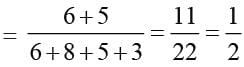Hence the probability of neither white nor black is 1/2.

Math Olympiad Test: Probability- 2 - Question 2

### A bag contains 5 red and some black balls. If the probability of drawing a black ball is thrice that of a red ball, the number of black balls in the bag is:

Detailed Solution for Math Olympiad Test: Probability- 2 - Question 2

Let the number of black balls in the bag be x
∴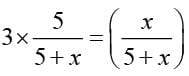⇒ x = 15
Hence, there are 15 blue balls in the bag.

Math Olympiad Test: Probability- 2 - Question 3

### A box contains 200 balls out of which 20 are defective. A bulb is drawn at random. What is the probability of drawing a non–defective bulb?

Detailed Solution for Math Olympiad Test: Probability- 2 - Question 3

P (getting a non–defective bulb)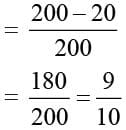Math Olympiad Test: Probability- 2 - Question 4

The sum of probabilities of all the outcomes of an experiment is:

Detailed Solution for Math Olympiad Test: Probability- 2 - Question 4

P (occurrence of a event) + P (non- occurrence of event) = total probability = 1

Math Olympiad Test: Probability- 2 - Question 5

A bag contains cards marked with numbers 51, 52, …., 100. A number is selected at random. What is the probability of getting a number which is not a multiple of 5?

Detailed Solution for Math Olympiad Test: Probability- 2 - Question 5

Number of multiples of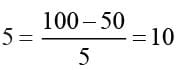∴  Required probability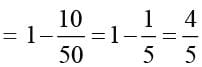Math Olympiad Test: Probability- 2 - Question 6

One card is drawn from a well–shuffled deck of 52 cards. The probability of drawing a red face card is:

Detailed Solution for Math Olympiad Test: Probability- 2 - Question 6

King, queen and jack are known as face cards
Number of red face cards = 2 × 3 = 6
∴ Required probability = 6/52 = 3/26

Math Olympiad Test: Probability- 2 - Question 7

Two dice are rolled simultaneously. Find the probability of getting their product as a odd number.

Detailed Solution for Math Olympiad Test: Probability- 2 - Question 7

The outcomes are:
(1, 1)  (1, 2)  (1, 3)  (1, 4)  (1, 5)  (1, 6)
(2, 1)  (2, 2)  (2, 3)  (2, 4)  (2, 5)  (2, 6)
(3, 1)  (3, 2)  (3, 3)  (3, 4)  (3, 5)  (3, 6)
(4, 1)  (4, 2)  (4, 3)  (4, 4)  (5, 5)  (5, 6)
(5, 1)  (5, 2)  (5, 3)  (5, 4)  (5, 5)  (5, 6)
(6, 1)  (6, 2)  (6, 3)  (6, 4)  (6, 5)  (6, 6)
Even × odd = even and odd × odd = odd.
∴ For product to be odd, both numbers should be odd.
∴ Required probability = 9/36 = 1/4.

Math Olympiad Test: Probability- 2 - Question 8

The probability of a certain event is:

Detailed Solution for Math Olympiad Test: Probability- 2 - Question 8

Probability defines the likelihood of occurrence of an event.
An event whose occurrence is 100% sure, those events are called certain events.
The probability of such an event is always 1.
Example: The probability of getting a number between 1 to 6 when rolling a die is a certain event.
Thus, the probability of an event that is certain to occur is 1.

Math Olympiad Test: Probability- 2 - Question 9

4 coins are tossed simultaneously. The probability of getting all tails is;

Detailed Solution for Math Olympiad Test: Probability- 2 - Question 9

The probability of getting all tails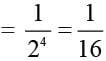Math Olympiad Test: Probability- 2 - Question 10

A dice is rolled 600 times and the occurrence of the outcomes are given below: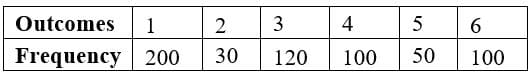The probability of getting a composite number is:

Detailed Solution for Math Olympiad Test: Probability- 2 - Question 10

P (composite number)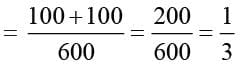{∵ composite numbers are 4 and 6}

Math Olympiad Test: Probability- 2 - Question 11

The unit place digit of 200 people’s mobile number is observed, and the following table is plotted: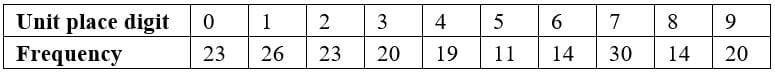A number is chosen at random. The probability that its unit place has prime number is:

Detailed Solution for Math Olympiad Test: Probability- 2 - Question 11

Prime numbers are 2, 3, 5, 7
∴ Sum of frequencies = 23 + 20 + 11 + 30 = 84
∴ Required probability = 84/200 = 42/100 = 0.42

Math Olympiad Test: Probability- 2 - Question 12

A dice is rolled twice. Find the probability of getting a prime number as a sum.

Detailed Solution for Math Olympiad Test: Probability- 2 - Question 12

Prime numbers which can get as a sum of the numbers on dice are 2, 3, 5, 7, 11
∴ Required probability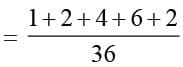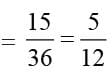Math Olympiad Test: Probability- 2 - Question 13

A coin is tossed 1000 times and the following frequencies are observed:
Head: 455, Tail: 545
The probability for getting tail is:

Detailed Solution for Math Olympiad Test: Probability- 2 - Question 13

Total outcomes = 1000
Frequency of Head = 455
Frequency of Tail = 545
Probability of getting head = 455/1000 = 0.455
Probability of getting tail = 545/1000 = 0.545 or 109/200.

Math Olympiad Test: Probability- 2 - Question 14

One card is drawn from a well-shuffled deck of 52 cards. What is the probability of getting a king?

Detailed Solution for Math Olympiad Test: Probability- 2 - Question 14

No. of cards in pack = 52
P (getting a king)
= 4/52 = 1/13.

Math Olympiad Test: Probability- 2 - Question 15

Three coins are tossed simultaneously. The probability of getting exactly 2 heads is:

Detailed Solution for Math Olympiad Test: Probability- 2 - Question 15

Formula: Probability = Number of favorable outcomes/Total number of outcomes
When three coins are tossed then the outcome will be any one of these combinations
(TTT, THT, TTH, THH, HTT, HHT, HTH, HHH)
So, the total number of outcomes is 8
Now, for exactly two heads, the favorable outcome is (THH, HHT, HTH)
We can say that the total number of favorable outcomes is 3
Again, from the formula
Probability = Number of favorable outcomes/Total number of outcomes
Probability = 3/8
∴ The probability of getting exactly two heads is 3/8.

## Mathematics Olympiad for Class 9

1 videos|43 tests
 Use Code STAYHOME200 and get INR 200 additional OFF Use Coupon Code
Information about Math Olympiad Test: Probability- 2 Page
In this test you can find the Exam questions for Math Olympiad Test: Probability- 2 solved & explained in the simplest way possible. Besides giving Questions and answers for Math Olympiad Test: Probability- 2, EduRev gives you an ample number of Online tests for practice

## Mathematics Olympiad for Class 9

1 videos|43 tests